## Completion Of Incomplete Pattern

#### Completion Of Incomplete Pattern

Direction: In each of the following questions, find out which of the answer figures (a), (b), (c) and (d) completes the figure?

1. Following Question has four alternatives, among which one completes the figures.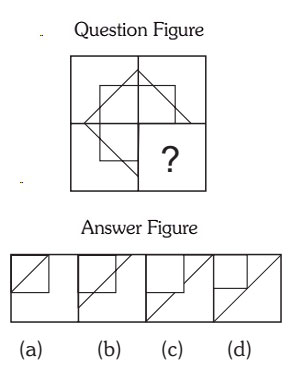1. complete the square and cross the line in middle.

##### Correct Option: B

`complete the square and cross the line in middle. Answer B will correct option to complete the figure.

1. Select the correct figure from the given Answer Figure that would complete the Question figure.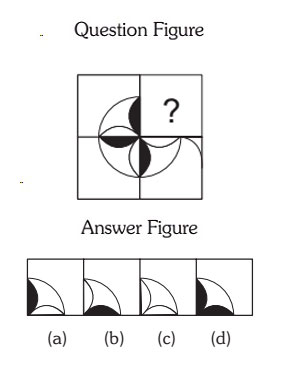1. Move the black figure 90 degree in clockwise direction.

##### Correct Option: B

Move the black figure 90 degree in clockwise direction.

1. Select the correct figure from the given Answer Figure that would complete the Question figure.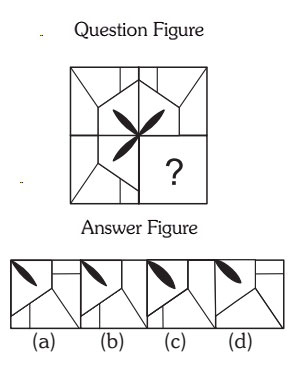##### Correct Option: C

1. Which one of the answer figures shall complete the given question figure?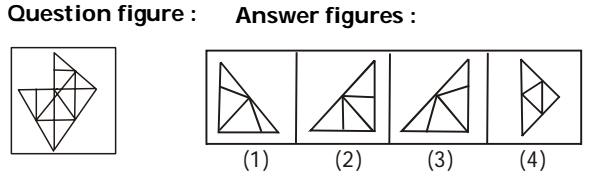1. NA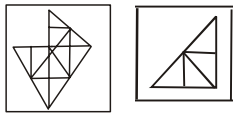##### Correct Option: B

NA1. In the given question, a part of the figure is missing. Select one of the response figures which exactly fits into the missing part of the question figure.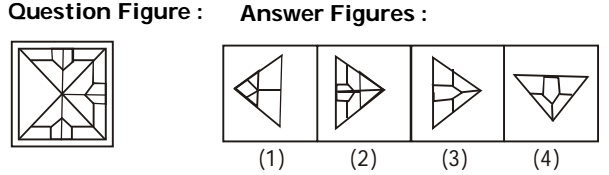1. NA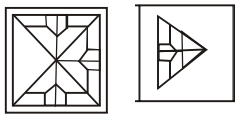##### Correct Option: B

NA